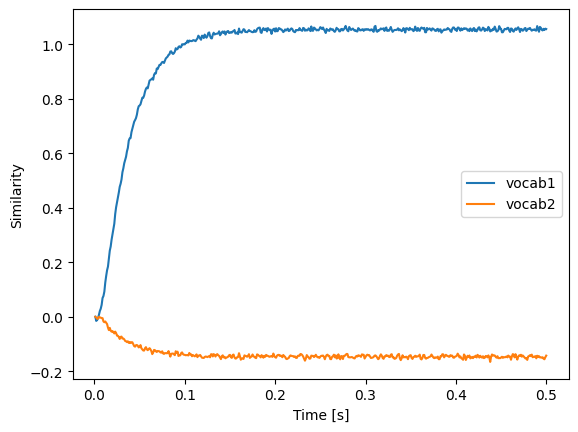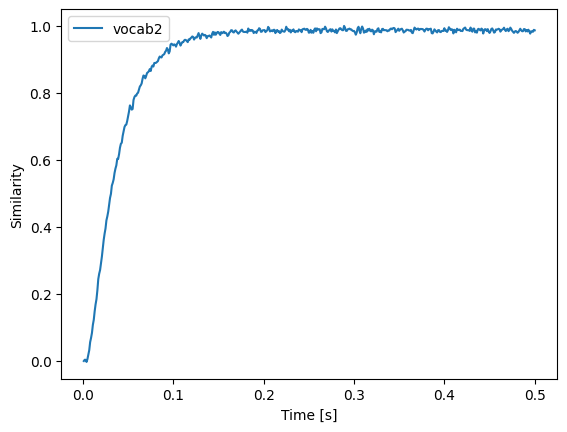Note

This documentation is for a development version. Click here for the latest stable release (v1.3.0).

# Vocabulary casting¶

Consider this SPA model:

:

%matplotlib inline

import matplotlib.pyplot as plt
import nengo
import numpy as np

import nengo_spa as spa

# Change the seed of this RNG to change the vocabulary
rng = np.random.RandomState(0)

:

d = 16
vocab1 = spa.Vocabulary(16, pointer_gen=rng)
vocab2 = spa.Vocabulary(16, pointer_gen=rng)

with spa.Network() as model:
state1 = spa.State(vocab=vocab1)
state2 = spa.State(vocab=vocab2)


In this model state1 and state2 are assigned to different and independent vocabularies. This means we cannot simply connect state1 and state2 in SPA actions because Nengo cannot know how to transform (“cast”) from one vocabulary to the other vocabulary. We are required to tell Nengo how we want to do this cast. Currently there two methods implemented.

## Reinterpretation¶

The reinterpret method can only be used with vocabularies of the same dimension. It will not change the semantic pointer passed from one state to the other, but changes it interpretation.

:

d1 = 16
d2 = 16
vocab1 = spa.Vocabulary(d1, pointer_gen=rng)
vocab1.populate("A")
vocab2 = spa.Vocabulary(d2, pointer_gen=rng)
vocab2.populate("A")

with spa.Network() as model:
state1 = spa.State(vocab=vocab1)
state2 = spa.State(vocab=vocab2)
spa.sym.A >> state1
spa.reinterpret(state1) >> state2

p = nengo.Probe(state2.output, synapse=0.03)

:

with nengo.Simulator(model) as sim:
sim.run(0.5)

:

plt.plot(sim.trange(), spa.similarity(sim.data[p], vocab1), label="vocab1")
plt.plot(sim.trange(), spa.similarity(sim.data[p], vocab2), label="vocab2")
plt.xlabel("Time [s]")
plt.ylabel("Similarity")
plt.legend(loc="best")

:

<matplotlib.legend.Legend at 0x7fdc97e592b0>## Translation¶

Translation matches the vocabularies by the keys and uses the outer product of the corresponding semantic pointers as a transformation matrix to transform vectors from one vocabulary to another vocabulary. This works also with vocabularies of differing dimensionality.

:

d1 = 16
d2 = 32
vocab1 = spa.Vocabulary(d1, pointer_gen=rng)
vocab1.populate("A")
vocab2 = spa.Vocabulary(d2, pointer_gen=rng)
vocab2.populate("A")

with spa.Network() as model:
state1 = spa.State(vocab=vocab1)
state2 = spa.State(vocab=vocab2)
spa.sym.A >> state1
spa.translate(state1, vocab2) >> state2

p = nengo.Probe(state2.output, synapse=0.03)

:

with nengo.Simulator(model) as sim:
sim.run(0.5)

:

plt.plot(sim.trange(), spa.similarity(sim.data[p], vocab2), label="vocab2")
plt.xlabel("Time [s]")
plt.ylabel("Similarity")
plt.legend(loc="best")

:

<matplotlib.legend.Legend at 0x7fdcd8f92820>## Further options¶

Both translate and reinterpret try to infer the target vocabularies, but this might not be possible in all cases. Thus, both functions take an additional argument which can either be a SPA module or a vocabulary. If it is a SPA module (e.g., translate(state1, state2)), that module’s vocabulary will be used as target vocabulary. If it is a vocabulary, the given vocabulary will be used as target vocabulary (e.g., translate(state1, vocab2)). Note that in the latter case a dictionary specifying the names and vocabularies has to be passed as the vocabs argument to the Actions instance like so: Actions('translate(state1, vocab2) -> state2').

Note that you can give the second argument to translate and reinterpret also as a keyword argument (e.g., translate(state1, vocab=state2).